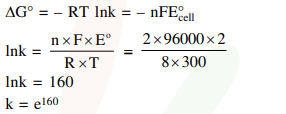# If the standard electrode potential for a cell is

Question:

If the standard electrode potential for a cell is $2 \mathrm{~V}$ at $300 \mathrm{~K}$, the equilibrium constant (K) for the reaction

$\mathrm{Zn}(\mathrm{s})+\mathrm{Cu}^{2+}(\mathrm{aq}) \rightleftharpoons \mathrm{Zn}^{2+}(\mathrm{aq})+\mathrm{Cu}(\mathrm{s})$

at $300 \mathrm{~K}$ is approximately.

$\left(\mathrm{R}=8 \mathrm{JK}^{-1} \mathrm{~mol}^{-1}, \mathrm{~F}=96000 \mathrm{C} \mathrm{mol}^{-1}\right)$

1. $e^{160}$

2. $e^{320}$

3. $e^{-160}$

4. $e^{-80}$

Correct Option: 1

Solution: